### Influence of Earth on Capacitance Calculation

In last article we discussed the technique for calculation of inductance and capacitance of double circuit transmission line, which can be easily applied for multi circuit lines. Of course before proceeding further I strongly recommend to go through those articles. I also advise to have a look at the two articles on calculation of line inductance and capacitance in general. Moreover as this article uses basic formulas of logarithm so we have tabulated few useful formulas at the bottom.

In the above three articles we developed some basic concepts. In the previous articles we neglected the influence of earth on capacitance calculation of transmission lines. But this is not true for Extra High voltage (EHV) and Ultra High Voltage (UHV) lines. For example for transmission lines above 200 kV voltage level the clearance distance between phases is quite comparable with the clearance between phase and ground. And electric field is considerably influenced by the presence of earth. This situation requires the consideration of influence of earth on calculation of line capacitance.

Here I will derive the formulas for calculation of transmission line capacitance.

Let us consider a charged line of circular cross section conductor as shown in Fig A. When this object is far removed from earth its electric field will be as shown in Fig-A(i), radial lines of force. In Fig-A(ii) is shown how the electric field is disturbed in the presence of Earth.  This field pattern above ground can be obtained by replacing the ground and placing an imaginary  oppositely charged similar conductor  same distance below the ground line. Here it is imagined that the ground level as a mirror. This is called image charge (see Fig-A (iii)). Now the problem is reduced to a pair of opposite charges.

Let us consider Fig-B where there are two very long line conductors of charge +q and -q coulmb per meter length of the conductor. From the basics it can be shown that the potential at a point P is vp
Then,

The above equation can also be written as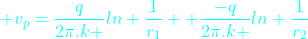The above equation can be imagined as the potential ( vp) at the point ' p ' is composed of two components one due to positive charge +q and other due to negative charge -q. These two are respectively first and second terms in the above equation.

Generalizing it we can say that the total potential at a point p due to several charges is equal to the sum of the potential due to all the individual charges.

Here next we will consider our favorite and practical case of the three phase line. Let us assume that the three phase conductors are placed in a general arbitrary form as shown in Fig -C. Now imagining the ground level as mirror we get the image charges which are -ve of actual charges on conductors as shown. Now the ground line can be removed. In the figure all the distances between the conductors and/or image are illustrated.

As illustrated in above case we will obtain the voltage difference between the conductors a and its image a' . For this we have to find the potential at the surface of a  and a'. Let the potential at a is va and the potential at a' is va'.

As already said the potential at the surface of conductor a is the simple algebraic sum of the potential due to charges on all the conductors including this particular conductor a. Similarly we can find the potential at the surface of a'.

This way we get,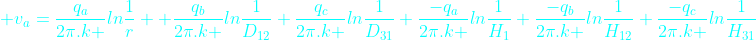Now Vaa' = va-va'

So from the above two equations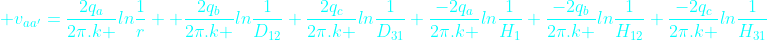Or in compact form we can write as

As already said the phase conductors are transposed. So for ⅓ rd of distance the conductor of  phase-a will take the position of  phase-b and conductor of phase-b will take that of  c .  So for all the three positions we will calculate the voltage for ' a ' phase and take the average. As already said in last articles  by transposition, the capacitance between any of the three conductors and neutral are made equal. If C denotes capacitance then after transposition we get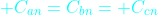Of course here we will concentrate on calculation of capacitance (Can) between phase-a  and neutral.

Due to transposition when the phase a conductors will take position of b as shown above and of course b will take position of c and c will take that of a. (You can think cyclically).

Similarly when  phase-a   conductor occupies the other position .

Let us call the average voltage between conductor a and image conductor a' be va-a'
Then,

Combining above three we get the value of va-a'
But we require the voltage from neutral (n) to phase-a conductor (see Fig-D), that is van

From Fig-D it is clear that,

Hence we get

Simplifying we get

or,

but the charges of three phases sum up to zero,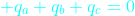So,

by rearranging the terms (see the properties of logarithm below at the end) we finally get

We know that the capacitance between phase a and neutral Can is,

So finally we get

From the above derived formula of capacitance Can  we observe that due to the consideration of influence of earth the denominator second term comes into picture and the capacitance Can  is more than what we would have got if earth influence was ignored.

Logarithm Formulas Table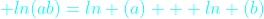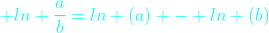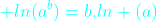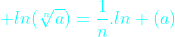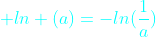Here ln means natural logarithm. Natural logarithm is the logarithm when the base is e. The value of e is approximately 2.718.

welcome to electrical world said...

complecatedzulfikar said...

but how with influence of earth on double circuit capcitance calculation???

MUNIB said...

Fantastic post.. Job well donesahishnu said...

whay is H1 in this case? is it distance of qa to -qa or qa to ground??Anonymous said...

Sir....
Use black color in place of light bluish color